# Blog Viewer

### The Role of Units Coordination in a Middle School Classroom (Part 3)

#### By Adrianne Burns posted 05-21-2018 08:07

Don’t you love when students have an “aha” moment?  What about your own “aha” moments? I still remember the first time I taught inequalities with algebra tiles and finally understood why the inequality symbol needs to be switched when multiplying or dividing by a negative number.

I have had many “aha” moments since being introduced to units coordination and the work of Anderson Norton and Amy Hackenberg through the US Math Recovery Council.  Incorporating this work into my classroom has been transformational for my thinking.  Here are just a few ways my thinking has shifted.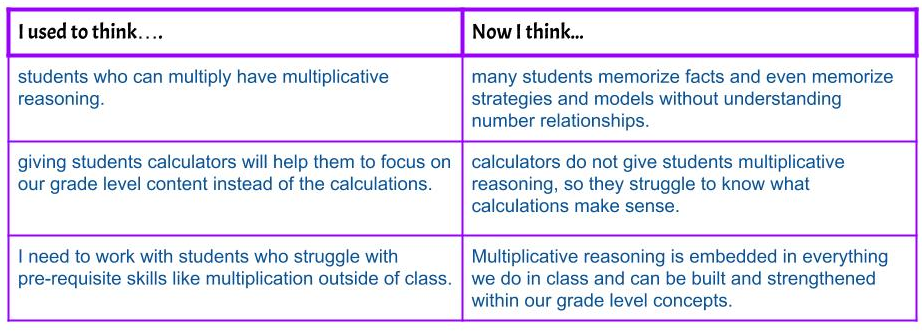The first blog post in this series introduces an assessment tool for units coordination, and the second post focuses on the role of units coordination in fractions. Units coordination, however, is present in most of the concepts we teach in middle school.  By identifying the levels of units coordination in a task, we can ask purposeful questions and select models and manipulatives that will strengthen students’ understanding of number relationships as well as give them access to the grade level content.

Much like fractions, working with percents can elicit the conversation about two wholes (seen in blog post 2).

A pair of shoes is on sale for \$100.  It is 60% off. What is the original price of the shoes?

Percents can be multipliers, and I was taught that “of” means “times”.  With this strategy (if I can even call it that), solving the equation 100 = 0.4x will give the original price.  Aside from lacking depth of understanding, this approach gives students who struggle with multiplicative reasoning no entry point to the task except to memorize steps.  If we instead look at this problem through the lens of units coordination, we can see there are at least 3 levels of units.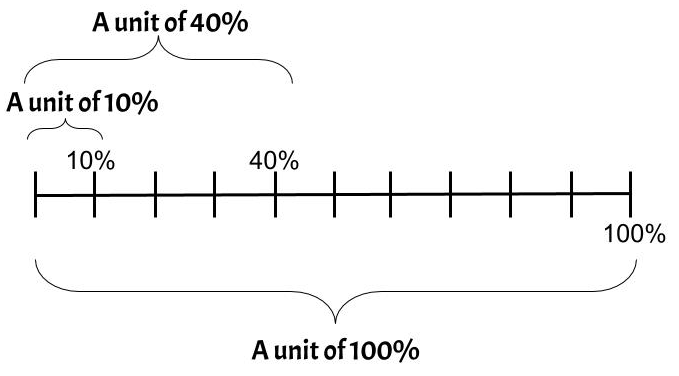Therefore, 40% can be thought of as the “new whole”.  Understanding that 40% is made up of 4 - 10% units can help students build their way to 100%.  Other students are able to build 100% with 2 units of 40% and a unit of 20% (or 2.5 units of 40%).  In this case, the number line is a far more powerful tool to put in the hands of students versus a calculator.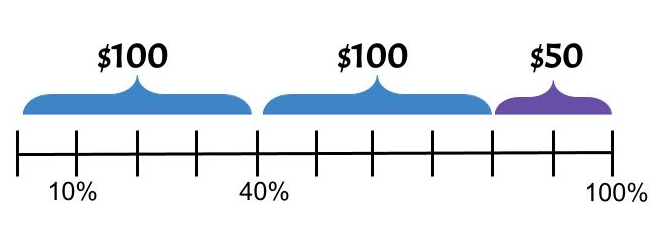This type of thinking can be extended into all proportional reasoning problems.  Think about the different units that exist in these number relationships.

50 pears cost \$25. How much would 75 pears cost?
Sarah takes 18 minutes to read 5 pages. How long does it take for her to read 30 pages?
It costs \$6 for ¾ pounds of sliced turkey.  What is the price per pound?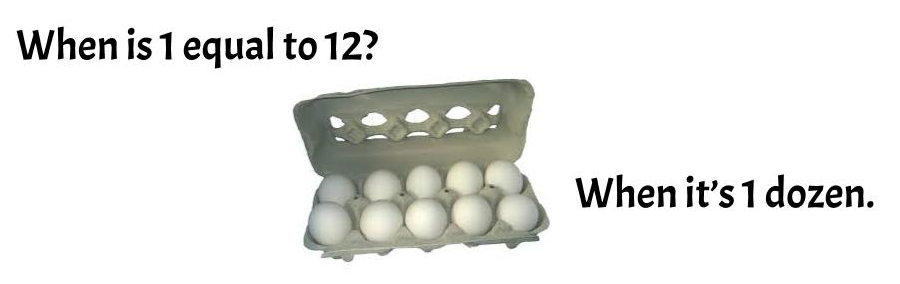This isn’t a bad math teacher joke.  It is the foundation of an “aha” moment I had this year when I discovered the role of units coordination in variables and solving algebraic equations.  I was working with a student using algebra tiles to solve an equation. She had gotten 7 x-tiles on one side of the
mat and 28 unit tiles on the other.  She was stuck and didn’t know where to go from there.

If 7 x-tiles equals 28, what would 1 x-tile be equal to?

I struggled to find questions to advance her thinking.  In the end, I showed her the division with tiles. Knowing where this student is in the stages of units coordination, I immediately knew the problem.  This student can only coordinate units through activity. This means she needs manipulatives that allow her to see the variable as 1 x at the same time as being 4 unit tiles, and the algebra tiles do not provide this.  Then I remembered this tweet: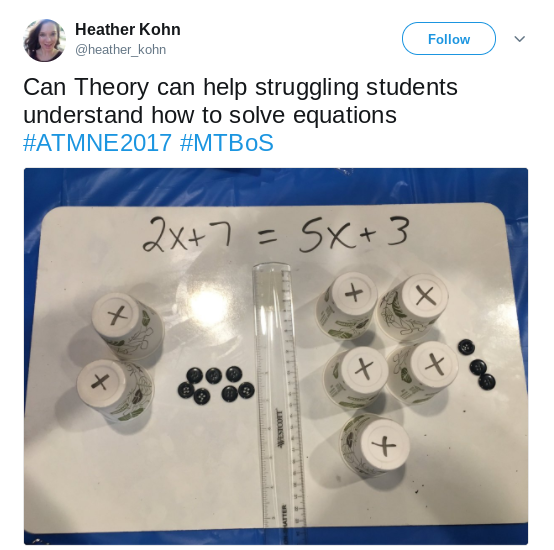Using cups and chips to solve equations allows students to see x as 1 cup as well as the number of units inside of it, much like the dozen eggs above.

As I stated earlier, units coordination is present in most of the concepts we teach.  Hopefully, with the few examples highlighted in this blog, you are able to look at the concepts you teach and the struggles your students have through the lens of units coordination.  What seem like calculation mistakes in the order of operations could be misunderstanding the units. The slope of a line is really the unit rate of its growth. As you notice the role of units coordination in the concepts you teach, please share them either in the comments or on Twitter at the hashtag #unitscoordination.

I look forward to continuing the conversation with you!